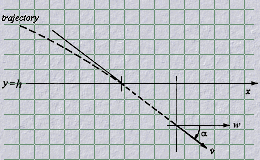### Aerodynamic Drag Estimation

The data set used in the "Parameter Estimation" example is not likely to be available in practical circumstances. However, a slight twist leads to a more efficient approach without the need for the intermediate trajectory data.

To make the estimation of the coefficient of drag more realistic let's add a bit of a tail wind and a single "measurement" of the impact distance, say, 2000 meters. Note that the wind is resolved into a velocity vector to obtain a true velocity with respect to wind which is used in computation of the aerodynamic drag force.In comparing the code with the "Parameter Estimation" case you'll note additional variation due to the replacement of trigonometric functions with their algebraic equivalents.

## Drag estimation code

``````
call optimal (iopt,isim,m,n,cd,range,tol,pif)
if(isim .eq. 1) call oprtn                 ! start simulation

if(mode() .eq. initialize) then
dist   = 0
height = 0
vx     = v0*cos(alfa)
vy     = v0*sin(alfa)
endif

*        Define & integrate eom
v     =  sqrt(vx**2 + vy**2)
vrw   =  v - wind*vx/v
drag  = .5*cd*ap*rho(height)*vrw**2/mass

dx(1) =  vx
dx(2) =  vy
dx(3) = -drag*vx/v
dx(4) = -drag*vy/v - g

call integral (mode(),dt(),4,S,id,dmy,dmy,x,dx)
*
if(height .lt. 0.) then                    ! reset on impact
isim = 0
call icrtn
xhit = dist - height*vx/vy
pif  = xhit - target
endif

``````